### How to use Si5351 #1 - Source Analysis

How to use Si5351 #1 - Source Analysis

Learn how to use the Si5351 for use with QRP radios include uBITX ,WSPR Beacone, Anatenna Anaylyser and many rf tools

As I am familiar with the AD9850, I first tried the Si5351 in uBITX. feel that using the Si5351 is suitable for QRP radios.

1.Si5351 is cheaper than the AD9850.
2.Si5351 outputs 3 or more clocks at the same time.
3.The Si5351 has two or more internal PLLs.
4.The AD9850 can generate waveforms, but the Si5351 only generates a clock.

We will analyze the famous sources using the si5351 and look at how they are used.

The preparation is the manual of the si5351 and the Si5351 module.

https://www.silabs.com/documents/public/data-sheets/Si5351-B.pdf
https://www.silabs.com/documents/public/application-notes/AN619.pdfThe Si5351 module is sold by Adafruit, Aliexpress, and qrplab. Alternatively, you can purchase the si5351 and connect it to the crystal and use
I used a module purchased from Aliexpress for about \$ 7. You can use any module.
You do not have to practice because it is not difficult.The Arduino nano and the Si5351 are only connected to the I2C and power.1.Si5351 Basic
SI5351 uses two PLLA and PLLB.
The PLL can generate clock from 600 MHz to 900 MHz.

2.How si5351 is used in uBITX
Use only one PLL.
PLL is used at 875Mhz.
Crystal uses 25Mhz.

In the initialization routine after system boot, set PLLA to generate 875Mhz clock.
CLK0, CLK1, and CLK2  use PLLA.

2.1 Init - Set PLLA to generate 875Mhz clock
Assign a 0 to register 149 // Spread Spectrum off

Assign a 0xFF to register 3 //all output DisabledAssign a 2 << 6 to register 183 //Set internal capacitor to 8pF
//note : By using an internal capacitor, the circuit can be simplified.Configuration for generating 875Mhz clock in PLLA

To generate the 875Mhz clock in the PLLA, calculate MSNA_P1 as shown in the manual.
The formula for MSNA_P1 is as follows.
MSx_P1[17:0] = 128 * a + Floor(128 * b /c) -512a is the divide value.
The clock we want is 875Mhz and the crystal uses 25Mhz, so the value of a is 35.
25Mhz * 35 = 875Mhz
Because MSNA_P1 alone can generate 875Mhz, P2 and P3 are not needed.MSx_P1[17:0] = 128 * 35 + 0 -512
msxpl = 128 * 35 - 512;
msxpl = 3968;

26 : 0  (MSNA_P3[15:8])
27 : 1  (MSNA_P3[7:0])
28 : msxpl >> 16 (MSNA_P1[17:16])
29 : msxpl >> 8  (MSNA_P1[15:8])
30 : msxpl (MSNA_P1[7:0])
31 : 0
32 : 0
33 : 0Assign 0x20 to Register 177 //Reset PLLA2.2 SetFreq (Using a PLLA operating at 875MHz, the generate desired clock)
Use the PLL frequency (875Mhz) to calculate ms, msb, and msc.

msa = si5351bx_vcoa / fout;       // Integer part of vco/fout
msb = si5351bx_vcoa % fout;      // Fractional part of vco/fout
msc = fout;                             // Divide by 2 till fits in reg

Ex) 30Mhz
msa = 875000000 / 30000000 = 29
msb = 875000000 % 30000000 = 5000000
msc = 30000000

The msc can input up to 20 bits, so if it exceeds, the value is decreased.

while (msc & 0xfff00000) {
msb = msb >> 1;
msc = msc >> 1;
}

Calculate msxp1.MSx_P1[17:0] = 128 * a + Floor(128 * b /c) -512
msxp1 = (128 * msa + 128 * msb / msc - 512) | (((uint32_t)si5351bx_rdiv) << 20);

Calculate msxp2.
msnx_p2[19:0] = 128 * b - c * floor(128 * b /c)

msxp2 = 128 * msb - 128 * msb / msc * msc; // msxp3 == msc;

Assign msxp1, msxp2, msxp3 to Register 42 + (clknum * 8)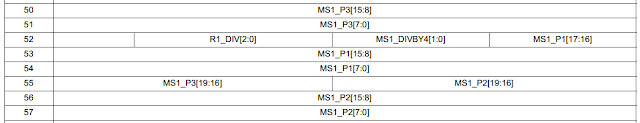Ex ) clk1
50 : ms1_p3 (msc) >> 8;
51 : ms1_p3 (msc)
52 : R1_DIV[2:0], MS1_DIVBY4[1:0], MS1_P1[17:16]   msxp1 >> 16
53 : msxp1 >> 8
54 : msxp1
55 : msxp3 (19:16), msxp2(19:16)
56 : msxp2 >> 8
57 ; msxp2

Assign Clock source (PLLA) and out power (2ma) to Register 16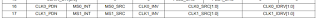0x0C | 0 (0:2ma, 1:4ma)

0x0C Register
00:XTAL
01:CLKIN
11:MultiSynth 0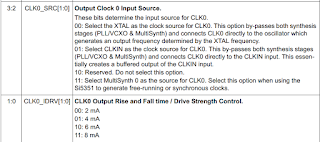--------------------------------------------------------------------------
2.3 List of used registers in uBITX Si5351 Module

Init function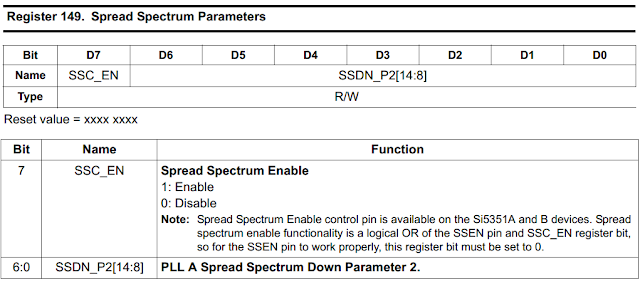3  //clock enable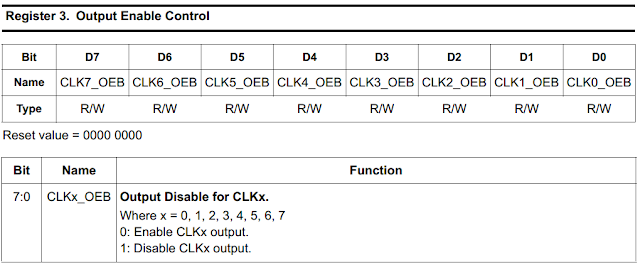183  //Crystal Capacitance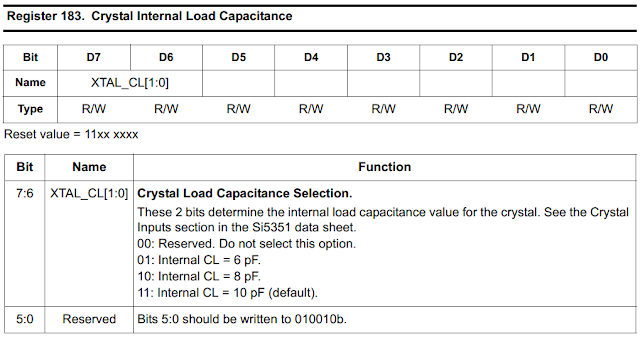26~33 : MSNA_P1~P3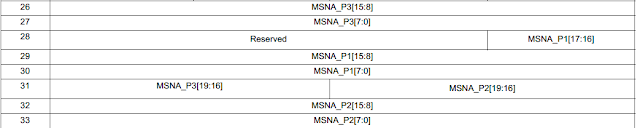177 : PLL ResetSet Frequency function
42~49, 50~57, 58~65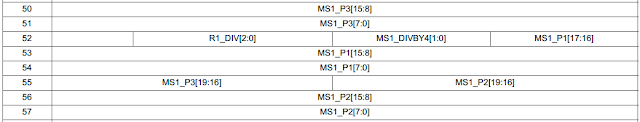16 : Clock0 control3  //clock enable

3   : clock enable
183 : crystal capacitance
26~33 : msna
177 : Pll reset

42~49 : MS1_P3 clock0
16 : clock0 control
3.G3ZIL Si5351 code
I needed more precise frequency control for the WSPR implementation.
so I implemented WSPR using the Etherkit Si5351 Library.  However, due to the large code size of the Etherkit Si5351, I had to give up some functions for uBITX.
Then I found the WSPR code of G3ZIL below.

https://qrp-labs.com/uarduino.html#g3zil
https://qrp-labs.com/images/uarduino/g3zil/G3ZIL_WSPR_Tx-Rx_V5.ino

The G3ZIL controls the frequency of the PLL to increase the accuracy of the output frequency.

In other words, Clock0 uses PLLA and Clock1 uses PLLB and changes frequency of PLLA or PLLB when frequency change is needed.

First, divide the VCO to obtain the desired frequency.
outdivider = 900000000 / frequency

If outdivider is greater than 900, continue to divide by 2. (Right shift)
Make outdivider an even number.

outdivider = 900000000 / frequency;
while (outdivider > 900){
R = R * 2;
outdivider = outdivider / 2;
}
if (outdivider % 2) outdivider--;

Calculate the PLL frequency to get the correct frequency.
fvco = outdivider * R * frequency;

Ex1) 1.5Mhz frequency
outdivider = 600, R = 1, fvco = 900,000,000

Ex2) 875Khz
outdivider = 1028 -> 514, R = 2, fvco = 899,500,000

Ex3) 876Khz
outdivider = 1027 -> 513 -> 512, R = 2, fvco = 897024000

Convert R to the format described in the Reference Guide.

switch (R){
case 1: R = 0; break;              // Bits [6:4] = 000
case 2: R = 16; break;             // Bits [6:4] = 001
case 4: R = 32; break;             // Bits [6:4] = 010
case 8: R = 48; break;             // Bits [6:4] = 011
case 16: R = 64; break;            // Bits [6:4] = 100
case 32: R = 80; break;            // Bits [6:4] = 101
case 64: R = 96; break;            // Bits [6:4] = 110
case 128: R = 112; break;          // Bits [6:4] = 111
}

We calculated the PLL to get the desired frequency more precisely.

#define c 1048574;                     // "c" part of Feedback-Multiplier from XTAL to PLL
1048574 = 1111 1111 1111 1111 1110

Calculate a, b, and c using the calculated PLL (fvco).

a = fvco / 25,000,000;

To reduce b to within 20 bits, perform the following operation.
f = fvco - a * F_XTAL;
f = f * c
f = f / 25,000,000
b = f

ex)in 876Khz case, a = 897024000 / 25,000,000 = 35
f = 897024000 - 875000000 = 22,024,000
f = 22,024,000 * 1048574 = 23093793776000;
f = 23093793776000 / 25,000,000 = 923751
b = f

Calcurate P1, P2, MSNA
P1 = 128 * outdivider - 512;

Calcurate PLL Register Value
MSN_P1 = 128 * A + (128 * b / c) - 512;
MSN_P2 = 128 * b / c;
MSN_P3 = c

Assign values to Register
- PLL
17 : (1 << 6) power down26 : MSN_P3 >> 8
27 : MSN_P3
28 : MSN_P1 >> 16 (MSNA_P1[17:16])
29 : MSN_P1 >> 8  (MSNA_P1[15:8])
30 : MSN_P1
31 : ((MSN_P3 & 983040) >> 12) | ((MSN_P2 & 983040) >> 16)
32 : MSN_P2 >> 8
33 : MSN_P2- Output clock
50 : 0;
51 : 1;
52 : ((MS0_P1 & 196608) >> 16) | R
53 : MS0_P1 >> 8
54 : MS0_P1
55 : 0
56 : 0
57 ; 0

//PLL RESET
177 : 32
17  : 0x4C;   //2ma

That is, this method is used when only one channel is used for one PLL. However, because of its high precision, WSPR signal generation is possible.

After creating the fundamental frequency as above, you can change the output frequency precisely by changing the value of P2.

void TX (unsigned long P2)                      // Changes TX frequency according to channel
{
Si5351a_Write_Reg (40, (P2 & 65280) >> 8);   // Bits [15:8]  of MSN_P2 in register 40
Si5351a_Write_Reg (41, P2 & 255);            // Bits [7:0]  of MSN_P2 in register 41
}

Test Code :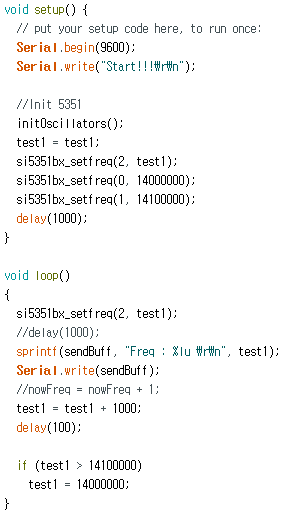ResultI have modified the code to be as concise as possible for testing purposes. You can download it from the link below
Source Code :

4.conclusion
I was trying to put the WSPR feature in uBITX. WSPR sources using the Si5351 were readily available on the Internet. Most of them used the Etherkit Si5351 Library.
The EtherKit Si5351 is a good library and can control up to two decimal places.

I was also able to easily add WSPR functionality to uBITX using the Etherkit 5351 library.
To use the Etherkit 5351 Library with uBITX, I had to remove some features because small storage size of Nano.

I thought I would modify the Si5351 Library for uBITX and gave my opinion to my friend Konstantinos (SV1ONW).
Konstantinos presented several ideas and I searched various Si5351 libraries.
And I could divide the various sources into two broad categories.

PLL fixed method and PLL dynamic method.
The PLL fixing method can use all the output channel provided by the si5351.
If you change the frequency by changing the PLL, you can increase the precision, but you can use only number of PLLs.

I am going to write code that can be used in a QRP transceiver by mixing the two methods.

1.Hello,

I've been studying the

https://qrp-labs.com/images/uarduino/g3zil/G3ZIL_WSPR_Tx-Rx_V5.ino

sketch and I'm interested in the precise frequency adjustment of the Si5351

in the code there is a constant integer declaration:

const int chan_mult[] ={384, 640, 896, 1280}; //20, 30, 40, 60

which is used to send the 162 bits of encoded symbols:

for (int i=0;i<sizeof(message)-1;i++)
{ // Now this is the message loop
lasttime = millis(); // Store away the time when the last message symbol was sent
channel = byte(message[i]); // Read channel out of message string
TX_P2 = TX_MSNB_P2 + chan_mult[band] * channel; // This represents the 1.46 Hz shift and is correct only for the bands specified in the array
TX(TX_P2); // TX at the appropriate channel frequency for....
while (millis() < lasttime + 683){} // .... 0,683 seconds
}

My question is : what is the formula to calculate these values for the WSPR bands?

384, 640, 896, 1280}; //20, 30, 40, 60

I'm assuming this is to create a 1.46 Hz spacing and it must be frequency dependant, but how would I get the all the correct values to use for the WSPR frequencies? ie:

http://wsprnet.org/drupal/node/7352

Thanks and hope to hear from you!

73 Jeff

### Release CEC Firmware v1.200 for uBITX All version(include V2, V3, V4, V5)

Release CEC Firmware v1.200 for uBITX All Version (include v2, v3, v4, v5) I did the firmware work for v5 when uBITX V5 was released, but I release it now. I received the feedback from a thankful beta tester and tested it myself by converting my uBITX v3 to v5 but I was not sure. I ordered the uBITX V5 and delivered the correct uBITX V5, so I made a little more fine-tuning. If you use V2, V3, V4, you do not need to update this firmware.